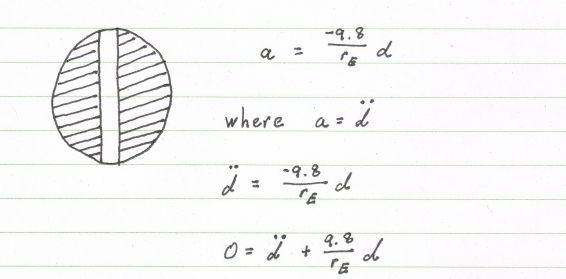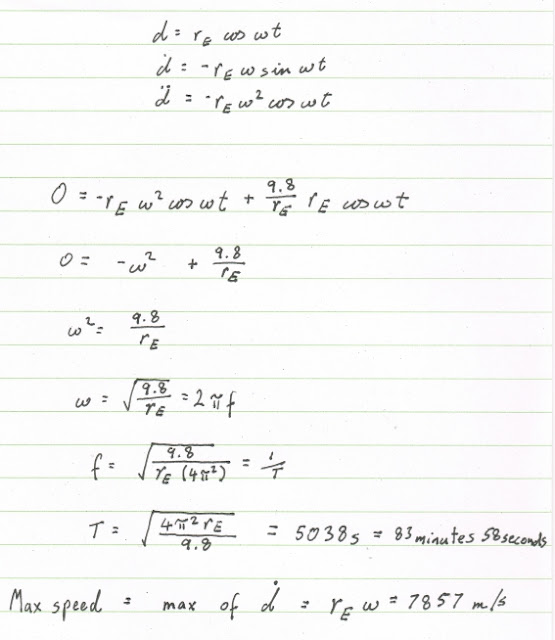## Friday, November 16, 2012

### I hope you find this blog enjoyable and helpful. Support it with a small donation.

By now everyone's probably seen Felix Baumgartner's high altitude parachute jump where he set a free fall speed record of mach 1.24.  The part of the footage that surprised me the most was how fast he disappeared after stepping off the platform.  After approximately 8 seconds he was just gone.  Thinking about the acceleration and speeds involved reminded me about the physics thought experiment called The Gravity Train.  If there was a tunnel the entire way through the earth and you jumped in what would happen?  How fast would you end up going?

First of all, ignore all the engineering challenges that make this impossible using any technology that we currently have.  There's no chance of this happening, this is one of those spherical chicken in a vacuum problems.  Then you need to know how the gravitational field inside the earth is orientated.  For this, we'll assume that the planet is spherical and has a constant density.  Which is way off, so just humour me.

We're all familiar with physics problems where the gravitational force between 2 planets is proportional to the inverse square of the distances between them, which is still true.  However, things get a little different once you start to get below the surface of a planet.  The equation for gravity doesn't change, the only difference is that you are now inside a distributed mass, so as well as being pulled down by the mass under you, there's mass above you pulling up.  Using The Shell Theorem, we know that the gravitational forces acting on a body inside a homogeneous shell of matter are zero.  They're all pulling in different directions and cancel each other out.  So the forces inside the planet depend only on the matter contained in the sphere underneath you.

The formula for acceleration on the planets surface is  GM / r^2.  As the radius changes linearly, the mass changes with the 3rd power, which is partially cancelled by the radius squared term, leaving the acceleration with a linear dependence on the radius.  This means at the centre of the planet the gravitational force experienced is 0, while at the surface it is 9.8 meters per second squared.Gravitational acceleration inside Earth

As you travel though the tunnel from one side of the planet to the other you would accelerate until you reached the centre, you would then start to slow down, and because of the symmetry of the problem you would reach the other side of the planet just as your velocity reached zero.  At this point you would start to fall again, which hints at a cosine term somewhere in the answer.  So let's throw some maths at this and see what numbers we can come up with.

The second derivative of displacement with respect to time is acceleration.  This can be placed into the formula for the acceleration inside Earth to give a differential equation to solve.Differential equation describing motion though Earth

This is an equation that will be familiar to many, it's not that hard and it describes quite a few oscillating systems.  It can be used to analyse the voltage in an LC circuit, or the displacement of a mass on a spring, among other uses.  It's useful to remember that the first derivative of displacement with respect to time is velocity.Calculating the coefficients

Now to work out the frequency and period of oscillation along with the maximum velocity by substituting back into our original differential equation.Final results

The period of the motion is around 84 minutes and is sinusoidal as suspected.  This would be for a round trip, so going from one side of the planet to the other would only take 42 minutes.  You would also reach a maximum speed of around 7850 m/second, or 28,000 km/hour, or 24 times the speed of sound.

It turns out that the 42 minute trip time is the same between any two points on the planets surface.  You don't have to go through the centre of the planet, and in the spirit of all "good" textbooks, I'll leave that as an exercise for the reader.

As an aside, 42 is a wonderful number, apart from "The Answer To the Ultimate Question of Life, The Universe, and Everything", the time in minutes a gravity train takes between two points on earth, the gyromagnetic ratio of a Hydrogen nucleus is 42.576 MHz/Telsa.  A useful fact if you do any work on MRI machines.

Why won't it work?

Now we've worked this out lets go through why it's completely useless.

First we would need a material for the tunnel walls that could withstand the tremendous heat and pressure at the centre of the planet.  It would also need to be able to deal with stresses induced by plate tectonics and the slight variation in the planets shape over time.  For this we would need to take a quick trip to Pandora to grab some of that Unobtainium that they have.

The tunnel would need to be evacuated.  If it wasn't, drag would cause objects to reach their terminal velocity and not reach the other side, eventually losing all kinetic energy and coming to rest in the centre of the planet.  Because of the large distances involved, there's a lot of atmosphere above the centre of the tunnel which would create a high air pressure meaning the drag would be even worse.  Just for fun, lets say drag didn't exist and we could reach 28,000 km/hour.  That's re-entry speed territory.  You would need a heat shield to prevent the object from burning up.

There is also another issue that I can't quite get a handle on.  If it was just a free-fall though the planet not using any sort of friction free rail system I think that the rotating Earth would rotate into the falling object.  I'm thinking Coriolis Effect. Maybe, maybe not.

Let's sum things up

It ain't going to happen.  No way, no how.  It's a good thing Felix had things like a parachute, and land to land on, otherwise he could be just floating around the Earth's core covered in energy drink advertisements.  So until we figure out how to build a Stargate, we'll have to be satisfied using planes to get around our planet.  How boring. :-(